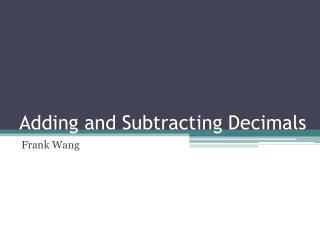DownloadDownload PresentationTélécharger la présentation- - - - - - - - - - - - - - - - - - - - - - - - - - - E N D - - - - - - - - - - - - - - - - - - - - - - - - - - -
##### Presentation Transcript

1. Adding and Subtracting Decimals Frank Wang

2. What and When do you Need to? When do you need to add and subtract decimals? The most common use of adding and subtracting decimals is money. Some other things that you will need to add and subtract is measuring, or just plain old math.

3. Decimal Place Value First, to add and subtract decimals, you will probably need to know the place values. The order is … TENTHS The trick is that every decimal place value is a normal place value but with a “ths” at the end. HUNDREDTHS THOUSANDTHS TEN THOUSANDTHS HUNDRED THOUSANDTHS MILLIONTHS You can consider the decimal point a “mirror”, just with a “ths” at the end of every reflection. (It goes on and on and on.)

4. Decimal Place Value What place values are these underlined decimals in? 0.74348 1.4675 0.38593 0.78439275943 0.734892075489302754903754893725840 _ _- _ _ _

5. Then What?? After you get the place values of the decimals correct, get the question and line up the place values. So, 15.923 + 28.78 becomes… 15.923 +28.780 The 0 is a placeholder so it lines up. Still, 28.78=28.780*. ___________ * Its like normal numbers but on the right end; 98=098 and so 28.78=28.780.

6. Solve! Add or subtract normally. Drop the decimal point. 15.923 +28.780 1 1 1 __________ 4 4. 7 0 3

7. Subtract! Same thing with subtraction. Follow the work shown.

8. Try it Out! Try this question to see if you learned how to do decimal addition correctly. 64.759 + 74.76 = ?????

9. The Answer Is…… 139.519!!!! Nice job if you got it right!! If you didn’t, try again. If you still got it wrong, follow the work.

10. Try it Out! Try out this question to see if you learned how to subtract decimals. 97.547 – 64.36 = ?????

11. The Answer is…… 33.187!!!! Nice job if you got it right!! If you didn’t, try again. If you still got it wrong, follow the work.

12. Review • What are the place values of decimals? • How do you solve a decimal problem? • When does decimal addition and subtraction come in handy?

13. Wooooo!! Congratulations! You have fully learned how to add and subtract decimals correctly. YAAAAAAAAAAAAAAAAY!!!!!!

14. Credits PowerPoint by Frank Wang Information from THE WORLD Typing by Frank Wang Wording by Frank Wang Contributors: YOU!!!!! Almost everything else by Frank Wang